# [SeNet] Squeeze-and-Excitation Networks （CVPR2018）

SeNet在channel 维进行了信息融合，是一种self_attention的实现。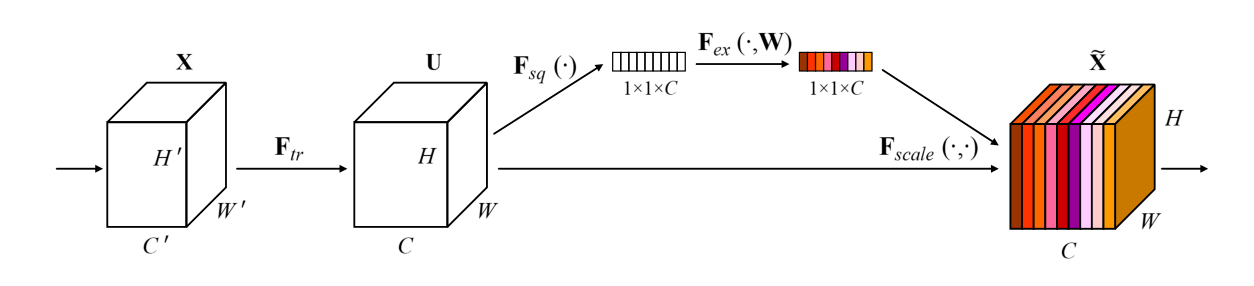1 × 1 × C 1\times1\times C 的tensor最终会通过sigmoid函数，表示每一维channel具有的信息的价值的差异，然后与原始张量channel维相乘。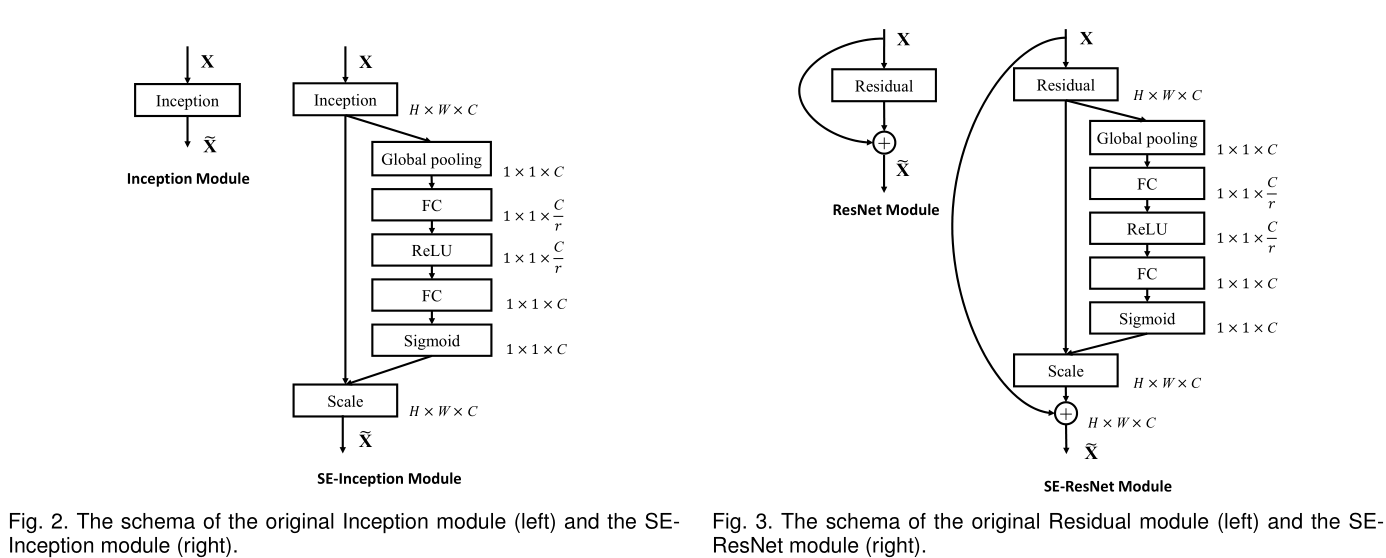SqueezeNoSqueeze
Global poolingNone
FC1 1 × 1 × C r 1\times1\times\frac{C}{r} Conv
FC2 1 × 1 × C 1\times1\times C Conv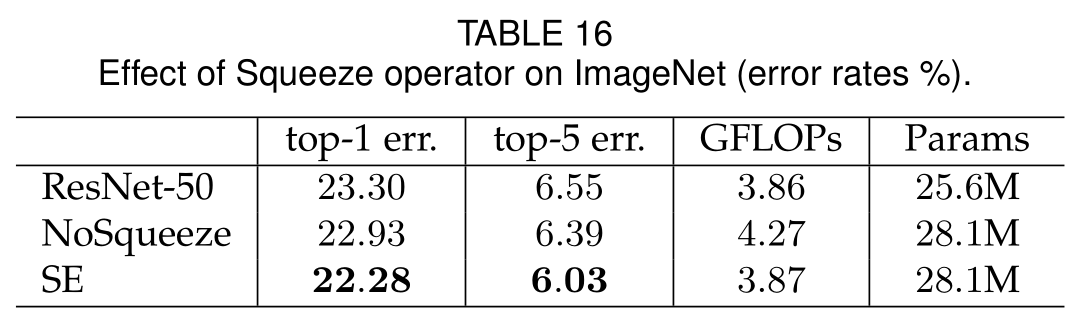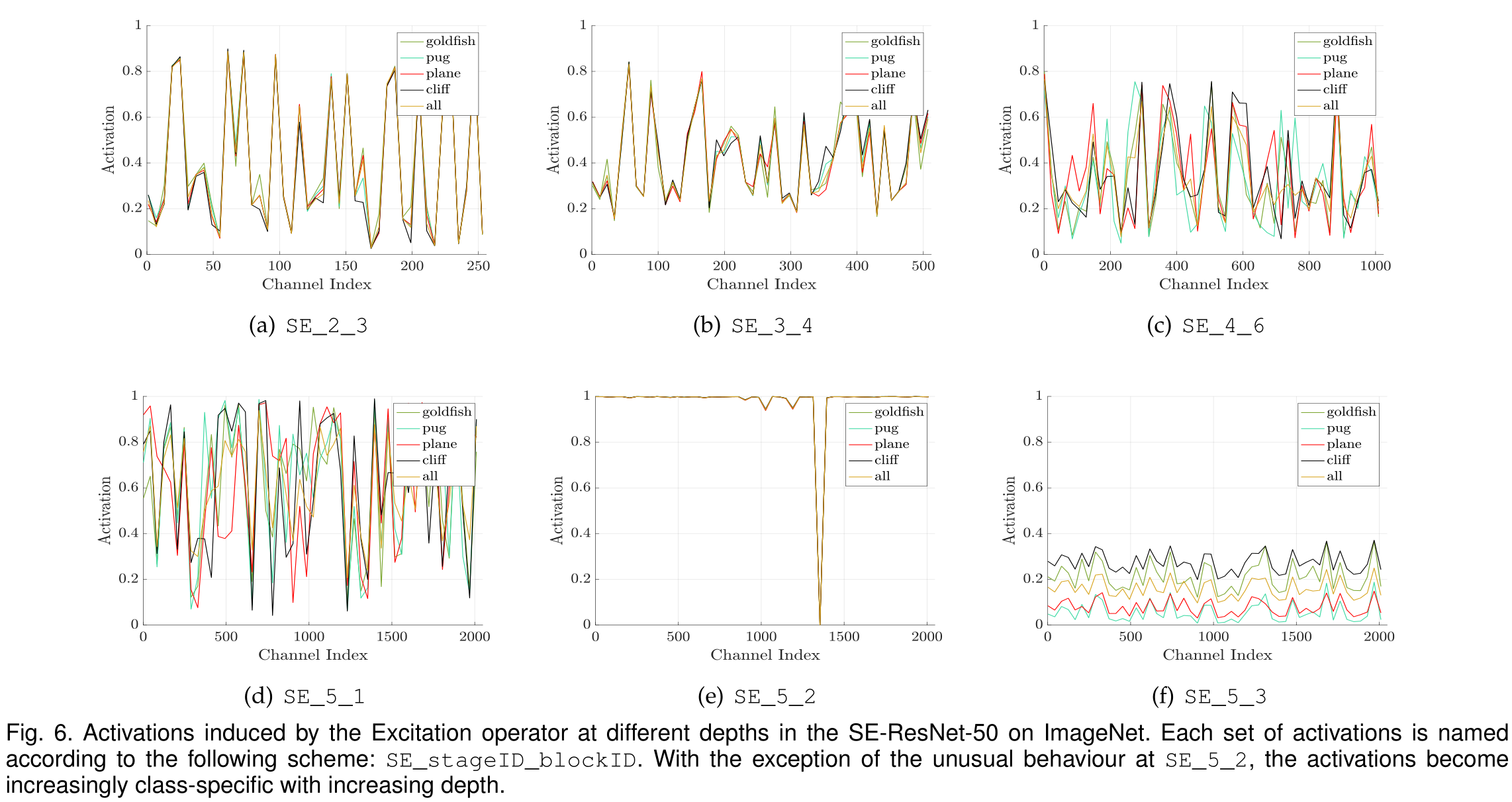1. 在浅层的网络中，不同类别的激活分布十分相似。表示在在此阶段，所有类别共享相同特征通道。早期的特征更具有一般性。
2. 随着网络变深，不同类别所对应的特征通道是不同。较深层的网络逐渐表现出差异性。
3. 在靠经网络输出的层，激励的效果趋向于饱和，表示最后的若干层对于不同类别所提供的差异性不如之前层重要。f 这张图之所以分布类似，偏重不同是因为之后需要输出分类类别。

https://blog.csdn.net/u014380165/article/details/78006626

# [Non-local] Non-local neural Networks （CVPR2018）

Non-local这一篇是在point-wise方面做attention的。def forward(self, x):
'''
:param x: (b, c, t, h, w)
:return:
'''

batch_size = x.size(0)

g_x = self.g(x).view(batch_size, self.inter_channels, -1)
g_x = g_x.permute(0, 2, 1)

theta_x = self.theta(x).view(batch_size, self.inter_channels, -1)
theta_x = theta_x.permute(0, 2, 1)
phi_x = self.phi(x).view(batch_size, self.inter_channels, -1)
f = torch.matmul(theta_x, phi_x)
f_div_C = F.softmax(f, dim=-1)

y = torch.matmul(f_div_C, g_x)
y = y.permute(0, 2, 1)
y = y.reshape(batch_size, self.inter_channels, *x.size()[2:])
W_y = self.W(y)
z = W_y + x

return z


# [GCNet] Non-local Networks Meet Squeeze-Excitation Networks and Beyond 2019-04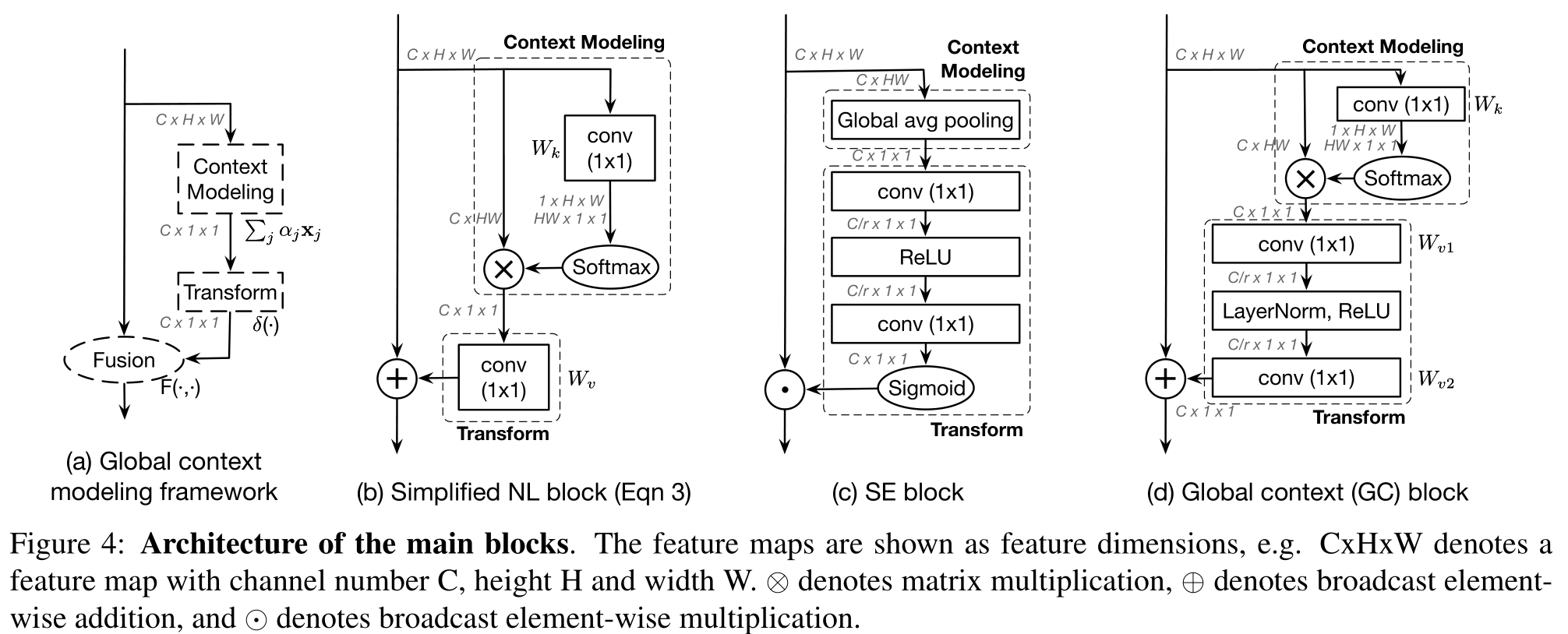11-094万+08-01876
09-201万+
09-28438
03-163万+
07-172626
04-248万+
05-21253
07-30622